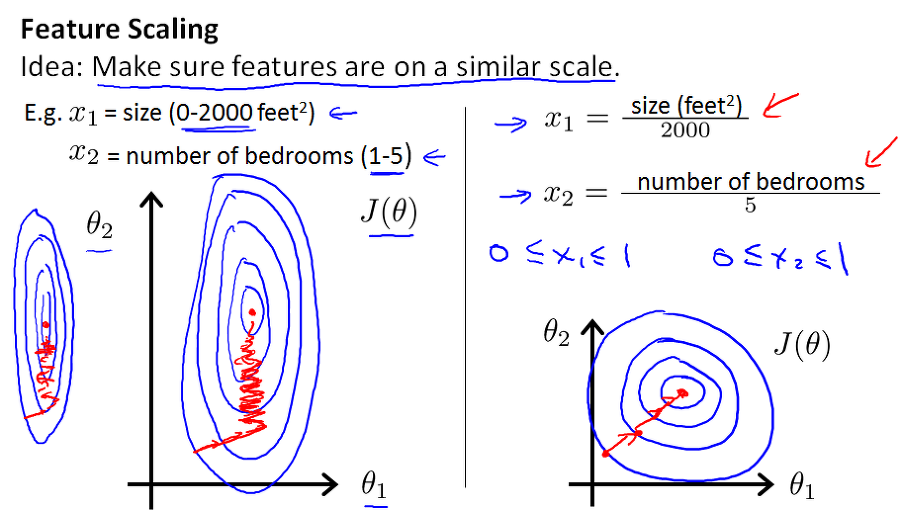# Feature Normalization

Categories:

Updated:

Normalizing features mean putting each of features in roughly the same range.

# Why Normalize Features?

We can speed up gradient descent by normalizing features. This is because $\theta$ will descend quickly on small ranges and slowly on large ranges. Theta will oscillate inefficiently down to the optimum when the variables are very uneven.# How?

Feature Normalization is composed of two steps.

## 1. Feature Scaling

Feature scaling means dividing the input values by the range (i.e. the maximum value minus the minimum value) of the input variables.

Commonly, we use ‘standard deviation’ as a ‘range’ parameter.

where $\overline {x_j}$ is the average of vector $x_j$.

## 2. Mean Normalization

Mean normalization involves subtracting the average value for an input variable from the values for that input variable, resulting in a new average value for the input variable of just zero.

## Resulting Formula

We combine these two steps and get following formula.

of just zero. To implement both of these techniques, adjust your input values as shown in this formula:

Categories: